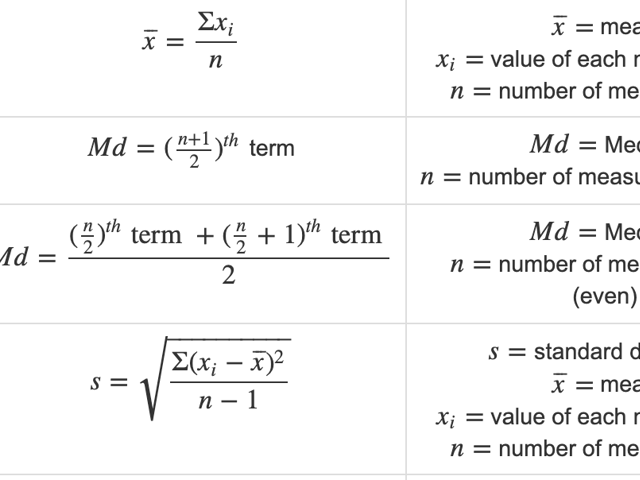# Formulas for Math on the ParaPro Test: Part 3—Data Analysis

Data analysis can be one of the trickiest parts of the Math section of the ParaPro Test. It requires not only math knowledge but the ability to choose the best strategy for solving each exercise.

Developing those skills requires practice, and thankfully, we’ve got you covered! In the following formula chart, you’ll find the essential formulas you’ll need for solving problems of a data analysis nature in the ParaPro Test.

Formulas for ParaPro Number Sense and Algebra Questions

Formulas for ParaPro Geometry and Measurement Questions

Then, use all three formula charts to solve the mathematics practice test problems at Union Test Prep.

## Data Analysis Math Formulas for the ParaPro Test

Category Formula Symbols Comment
Data Analysis $$Du=Su\cdot \dfrac{Du}{Su} = Su \cdot CF$$ $$Du =$$ Desired Unit
$$Su =$$ Starting Unit
$$CF =$$ Conversion Factor
Multiple Steps may
be needed
Data Analysis $$a\cdot b\% = a\cdot \dfrac{b}{100}$$ $$a =$$ any real number
$$b\% =$$ any percent
Remember to
simplify (if possible)
Data Analysis $$\% = \dfrac{\lvert b-a \rvert}{b} \cdot 100 = \dfrac{c}{b} \cdot 100$$ $$\% = \%$$ increase or decrease
$$a =$$ new value
$$b =$$ original value
$$c =$$ amount of change

Data Analysis $$\overline{x} = \dfrac{ \Sigma x_i}{n}$$ $$\overline{x} =$$ mean
$$x_i =$$ value of each measurement
$$n =$$ number of measurements

Data Analysis $$Md = (\frac{n+1}{2})^{th}$$ term $$Md =$$ Median
$$n =$$ number of measurements (odd)

Data Analysis $$Md = \dfrac{(\frac{n}{2})^{th} \text{ term } + (\frac{n}{2} + 1)^{th} \text{ term }}{2}$$ $$Md =$$ Median
$$n =$$ number of measurements (even)

Data Analysis $$s = \sqrt{\dfrac{\Sigma (x_i - \overline {x})^2}{n-1}}$$ $$s =$$ standard deviation
$$\overline{x} =$$ mean
$$x_i =$$ value of each measurement
$$n =$$ number of measurements

Data Analysis $$V = s^2$$ $$V =$$ Variance
$$s =$$ standard deviation

Data Analysis $$CV = RSD = 100 \cdot s \sqrt{x}$$ $$CV =$$ Coefficient of variation
$$RSD =$$ Relative standard deviation
$$s =$$ standard deviation

Data Analysis $$y = mx + b$$ $$m =$$ slope
$$b =$$ y-intercept
$$y =$$ dependent variable
$$x =$$ independent variable